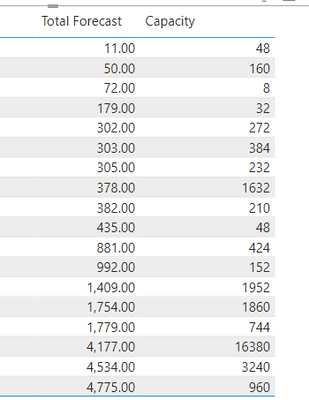cancel
Showing results for
Did you mean:Regular Visitor

## Calculating in visualisation

Hey,

I want to calculate with the data in the table in 'Report'. The data in the table is grouped by through the visualisation, so I can't use the data. How can I use the data in the table to calculate a new column?I want a new column 'Accuracy'. This through 'Capacity' divided by 'Total Forecast'. In conclusion I am not able to use this in the data.

11 REPLIES 11Regular Visitor

Hey,

I want to calculate with the data in the table in 'Report'. The data in the table is grouped by through the visualisation, so I can't use the data. How can I use the data in the table to calculate a new column?I want a new column 'Accuracy'. This through 'Capacity' minused by 'Total Forecast'. In conclusion I am not able to use this in the data.Community Champion

@melih what do you mean you are not able to?
How does the desired report looks like?
Did you try to write this measure:
Accuracy = SUM('Table'[Capacity]) - SUM('Table'[Total Forecast])Showcase Report – Contoso By SpartaBIRegular Visitor

Didn't work man. The result needs to be 48-11 = 37 and not 15. Do you got another idea?Community Champion

Capacity and total forcast are measures?Regular VisitorCommunity Champion

ok, so thery are columms and you did implicit measures on them.
How are they aggregated in you visual? SUM / AVERAGE?
If it's not just sum then you need to create explicit measures for them and them just reduce 1 measure from the otherRegular Visitor

HEy @selimovd ,

Thank you. Actually I would like to minuse them. How can I fix that?Super User

Hey @melih ,

as far as I would guess, the subtraction has to happen on a row level in the table. For that reason you have to use SUMX, which iterates over each row.

The following measure would go row by row and subtract forecast from capacity and build a sum at the end:

``Accuracy = SUMX ( myTable, myTable[Capacity] - myTable[Total Forecast] )``

Is that what you need?

If not, could you give me an example how the result should look like?

If you need any help please let me know.
If I answered your question I would be happy if you could mark my post as a solution ✔️ and give it a thumbs up 👍

Best regards
Denis

Blog: WhatTheFact.biRegular Visitor

Hey @selimovd ,

It didn't work. For example the first result of the row gives me 15. But 48-11 should be 37.Super User

Hey @melih ,

so you don't want it on a row level 😉

In that case you can just subtract:

``Accuracy = SUM ( myTable[Capacity] ) - SUM ( myTable[Total Forecast] )``

If you need any help please let me know.
If I answered your question I would be happy if you could mark my post as a solution ✔️ and give it a thumbs up 👍

Best regards
Denis

Blog: WhatTheFact.biSuper User

Hey @melih ,

to add a "new column" to the visual, you have to create a measure and add it to the visual.

The measure is then executed in every row with the context of the row.

The following measure should do the job:

``Accuracy = DIVIDE ( SUM ( myTable[Capacity] ), SUM ( myTable[Total Forecast] ) )``

If you need any help please let me know.
If I answered your question I would be happy if you could mark my post as a solution ✔️ and give it a thumbs up 👍

Best regards
Denis

Blog: WhatTheFact.bi